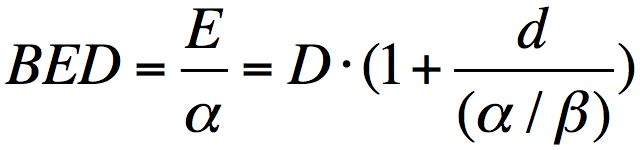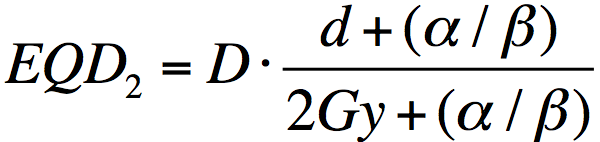ON OFF

A fractionation schedule of D Gy in n fractions (with d Gy per fraction) is equivalent to a BED of BED Gy10 and EQD2 of EQD2 Gy, assuming an α / β ratio of a/b.

## What is RBApp?

Keep in mind that the results of calculations are for educational purposes only, and should not be used for clinical decision making.

## Directions

To use this program, input numbers into two of three of the fields: Total dose, number of fractions, dose per fraction. The program will automatically calculate the third value.

A alpha/beta ratio is also required. Classically, a value of α / β = 10 is used for tumours and α / β = 3 for normal tissues.

## Known Issues

If input values are invalid, an alert dialog box will display and the program will take users to a blank Results screen. Users need to click the top left "RBApp" button to return to the main screen to re-enter their values. (July 2012)

Clicking on an external link on iOS devices will lead users away from RBApp, and a user may need to click the Home button to return to the main screen. (July 2012)

RBApp

## Basic Mode

The default mode allows you to calculate BED and EQD2 from knowledge of the following parameters:

• two of three of the following variables:
• total dose (D)
• number of fractions (n)
• dose per fraction (d)
• α / β ratio

The advanced mode will allow you to calculate all values from knowledge of at least three of the following parameters:

• BED or EQD2
• α / β ratio
• one of three of the following variables:
• total dose (D)
• number of fractions (n)
• dose per fraction (d)BED = biologically effective dose
EQD2 = dose in 2 Gy fractions biologically equivalent to dose D given in fractions of size d, in units of Gray

E is biological effect, D is total dose (D = nd), d is dose per fraction, n is the number of fractions, and alpha/beta is a property of the irradiated tissue.